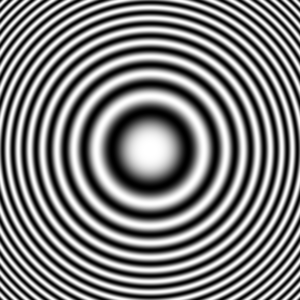# Square (algebra) facts for kids

Kids Encyclopedia Facts
(Redirected from Squared)Fresnel's zone plates have rings with equally spaced squared distances to the center

In mathematics, a square of a number means the result of multiplying the number by itself. For example, the square of 6 is 6×6 = 36.

Squaring a number can also be written as 62 = 36.

Many of the units of area are squares. For example, a square meter means the area equal to the area of a rectangle which is one meter tall and one meter wide.

## Related pagesSquare (algebra) Facts for Kids. Kiddle Encyclopedia.